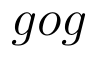Select Page

# Maths Determinant CBSE 12 Science Answers for MCQ in English

Maths Determinant CBSE 12 Science Answers for MCQ in English to enable students to get Answers in a narrative video format for the specific question.

Expert Teacher provides Maths Determinant CBSE 12 Science Answers for MCQ through Video Answers in English language. This video solution will be useful for students to understand how to write an answer in exam in order to score more marks. This teacher uses a narrative style for a question from Determinant not only to explain the proper method of answering question, but deriving right answer too.

Please find the question below and view the Answer in a narrative video format.

Question:

## Similar Questions from CBSE, 12th Science, Maths, Determinant

Question 1 : Find the inverse of the matrix. (View Answer Video)

Question 2 : Evaluate  the determinants in :. (View Answer Video)

Question 3 : Let A be square matrix of order 3 * 3, then |kA|is equal to (View Answer Video)

Question 4 :  Find the area of the triangle with vertices at the points (-2, -3), (3, 2), (-1, -8). (View Answer Video)

Question 5 :  Evaluate the determinants:. (View Answer Video)

### Relations and Functions

Question 1 :be  defined as f(x) =(View Answer Video)

Question 2 : Functionsare defined respectively, by, find(View Answer Video)

Question 3 :  A functionis surjective if and only if , (View Answer Video)

Question 4 : Let A = {1, 2, 3}. Then, number of equivalence relations containing (1, 2) are, (View Answer Video)

Question 5 : The number of binary operations which can be defined on the setis, (View Answer Video)

### Application of Derivatives

Question 1 : The length x of a rectangle is decreasing at the rate of 5cm/minute. and width y is increasing at the rate of 4cm/minute. When x=8cm and y=6 cm, find the rate of changes of:

Question 2 : The volume of a cube is increasing at the rate of 9 cubic centimetres per second. How fast is the surface area increasing when the length of an edge is 10 centimetres? (View Answer Video)

Question 3 : On which of the following intervals in the functionstrictly decreasing? (View Answer Video)

Question 4 : What is the maximum value of the function sin x+ cos x? (View Answer Video)

Question 5 : For all real values of x, the minimum value ofis  (View Answer Video)

### Vector Algebra

Question 1 : Find the direction of the vector(View Answer Video)

Question 2 : Find the area of the triangle with vertices A(1, 1, 2), B(2, 3, 5) and C(1, 5, 5). (View Answer Video)

Question 3 : If the vertices A, B, C of a triangle ABC have position vectors (1, 2, 3), (-1, 0, 0), (0, 1, 2) respectively then find <ABC (<ABC is the angle between the vectors BA and BC). (View Answer Video)

Question 4 :  Write a unit vector in the direction of the sum of vectorsand(View Answer Video)

Question 5 : Find a vector in the direction of vectorwhich has magnitude 8 unit. (View Answer Video)